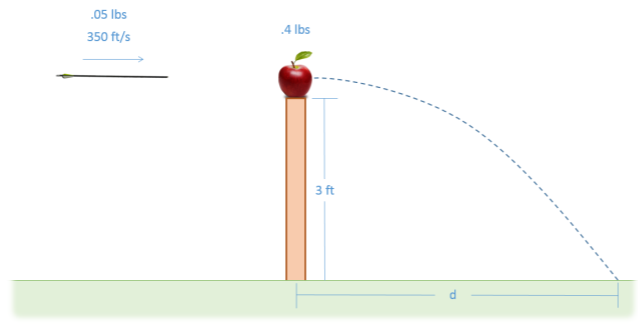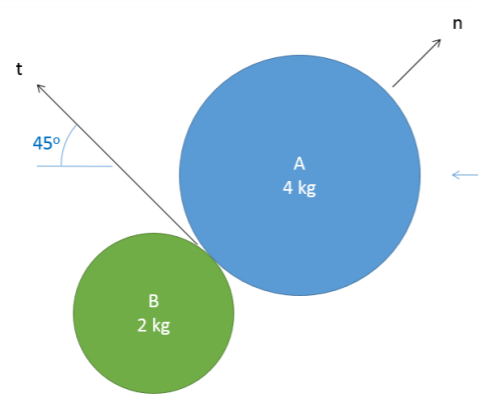# 10.6: Chapter 10 Homework Problems

$$\newcommand{\vecs}{\overset { \rightharpoonup} {\mathbf{#1}} }$$ $$\newcommand{\vecd}{\overset{-\!-\!\rightharpoonup}{\vphantom{a}\smash {#1}}}$$$$\newcommand{\id}{\mathrm{id}}$$ $$\newcommand{\Span}{\mathrm{span}}$$ $$\newcommand{\kernel}{\mathrm{null}\,}$$ $$\newcommand{\range}{\mathrm{range}\,}$$ $$\newcommand{\RealPart}{\mathrm{Re}}$$ $$\newcommand{\ImaginaryPart}{\mathrm{Im}}$$ $$\newcommand{\Argument}{\mathrm{Arg}}$$ $$\newcommand{\norm}{\| #1 \|}$$ $$\newcommand{\inner}{\langle #1, #2 \rangle}$$ $$\newcommand{\Span}{\mathrm{span}}$$ $$\newcommand{\id}{\mathrm{id}}$$ $$\newcommand{\Span}{\mathrm{span}}$$ $$\newcommand{\kernel}{\mathrm{null}\,}$$ $$\newcommand{\range}{\mathrm{range}\,}$$ $$\newcommand{\RealPart}{\mathrm{Re}}$$ $$\newcommand{\ImaginaryPart}{\mathrm{Im}}$$ $$\newcommand{\Argument}{\mathrm{Arg}}$$ $$\newcommand{\norm}{\| #1 \|}$$ $$\newcommand{\inner}{\langle #1, #2 \rangle}$$ $$\newcommand{\Span}{\mathrm{span}}$$$$\newcommand{\AA}{\unicode[.8,0]{x212B}}$$

Exercise $$\PageIndex{1}$$

A jackhammer exerts the impulse shown below on the 1.5-kilogram bit to drive it towards the ground. If the bit starts at rest, what will the expected velocity of the bit be at the end of the impulse?Figure $$\PageIndex{1}$$: problem diagram for Exercise $$\PageIndex{1}$$. Graph of the force exerted by the jackhammer on its bit as a function of time.
Solution

$$v = 90 \, m/s$$

Exercise $$\PageIndex{2}$$

A 0.05-lb arrow traveling at 350 ft/s impacts a 0.4-lb apple on the top of a post that is 3 feet tall. If the arrow becomes lodged in the apple, how far would we expect the apple to travel $$(d)$$ before hitting the ground?Figure $$\PageIndex{2}$$: problem diagram for Exercise $$\PageIndex{2}$$. An arrow is about to hit an apple; the two will fall together off the pole where the apple is balanced.
Solution

$$d = 16.8 \, ft$$

Exercise $$\PageIndex{3}$$

A basketball impacts a metal surface as shown below. If the initial velocity of the basketball was 3 ft/s straight down and the coefficient of restitution is 0.85, what is the expected speed and direction $$(\theta)$$ of the ball after the impact?Figure $$\PageIndex{3}$$: problem diagram for Exercise $$\PageIndex{3}$$. A basketball falls onto a metal surface tilted at 25° from the horizontal, bouncing off at some speed at an angle of $$\theta$$ from the horizontal.
Solution

$$v = 2.64 \, ft, \, \theta = 36.25°$$

Exercise $$\PageIndex{4}$$

Puck A, traveling with an initial velocity of 5 m/s, strikes the stationary Puck B. Assuming the collision is elastic, what will the velocity of each puck be immediately after the collision?Figure $$\PageIndex{4}$$: problem diagram for Exercise $$\PageIndex{4}$$. Puck A moves towards the left, striking the upper right corner of Puck B and creating a plane of contact that translates to a tangential axis 45° from the horizontal.
Solution

$$\vec{v}_{A, f} = [-3.34, 1.67] \, m/s$$

$$\vec{v}_{B, f} = [-3.34, -3.34] \, m/s$$

Exercise $$\PageIndex{5}$$

A jet engine with a mass of 700 kg and an air mass flow rate of 50 kg/s is mounted to a stand as shown below (a set of legs on each side, only one half shown). Based on the input and output velocities shown below, determine the thrust force of the engine and the forces in stand members $$AB$$, $$AD$$, and $$CD$$. Be sure to indicate if each member is in tension or compression.Figure $$\PageIndex{5}$$: problem diagram for Exercise $$\PageIndex{5}$$. A jet engine facing towards the left is mounted on a stand consisting of 4 legs, two on the side of the engine facing the viewer and two on the opposite side, with a single diagonal member connecting the legs within each set.
Solution

$$F_{thrust} = 26 \, kN$$

$$F_{AB} = 6.04 \, kN \, T$$

$$F_{AD} = 15.01 \, kN \, C$$

$$F_{CD} = 1.96 \, kN \, C$$

This page titled 10.6: Chapter 10 Homework Problems is shared under a CC BY-SA 4.0 license and was authored, remixed, and/or curated by Jacob Moore & Contributors (Mechanics Map) via source content that was edited to the style and standards of the LibreTexts platform; a detailed edit history is available upon request.